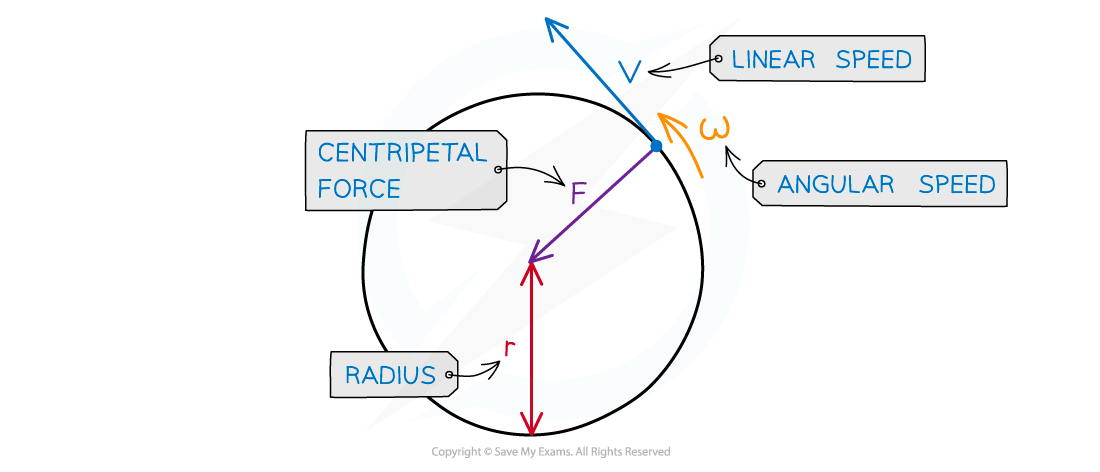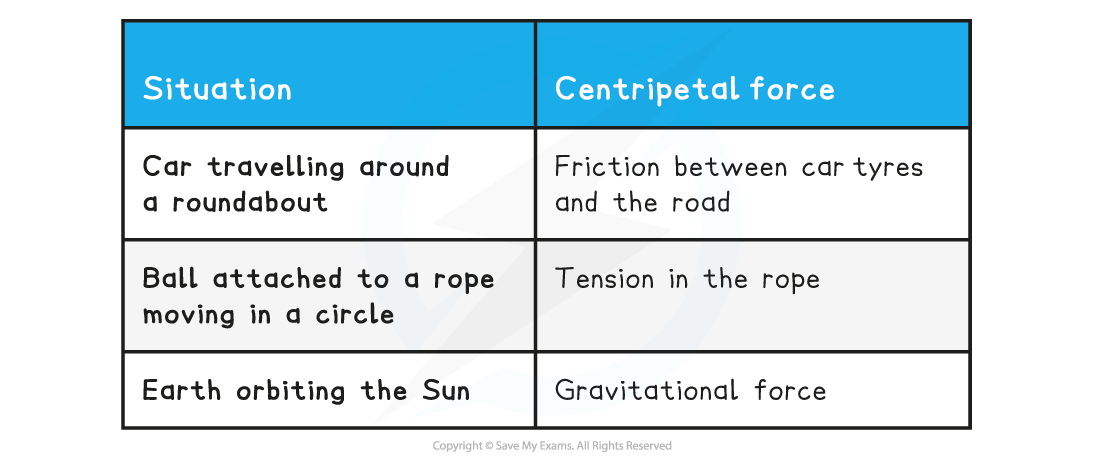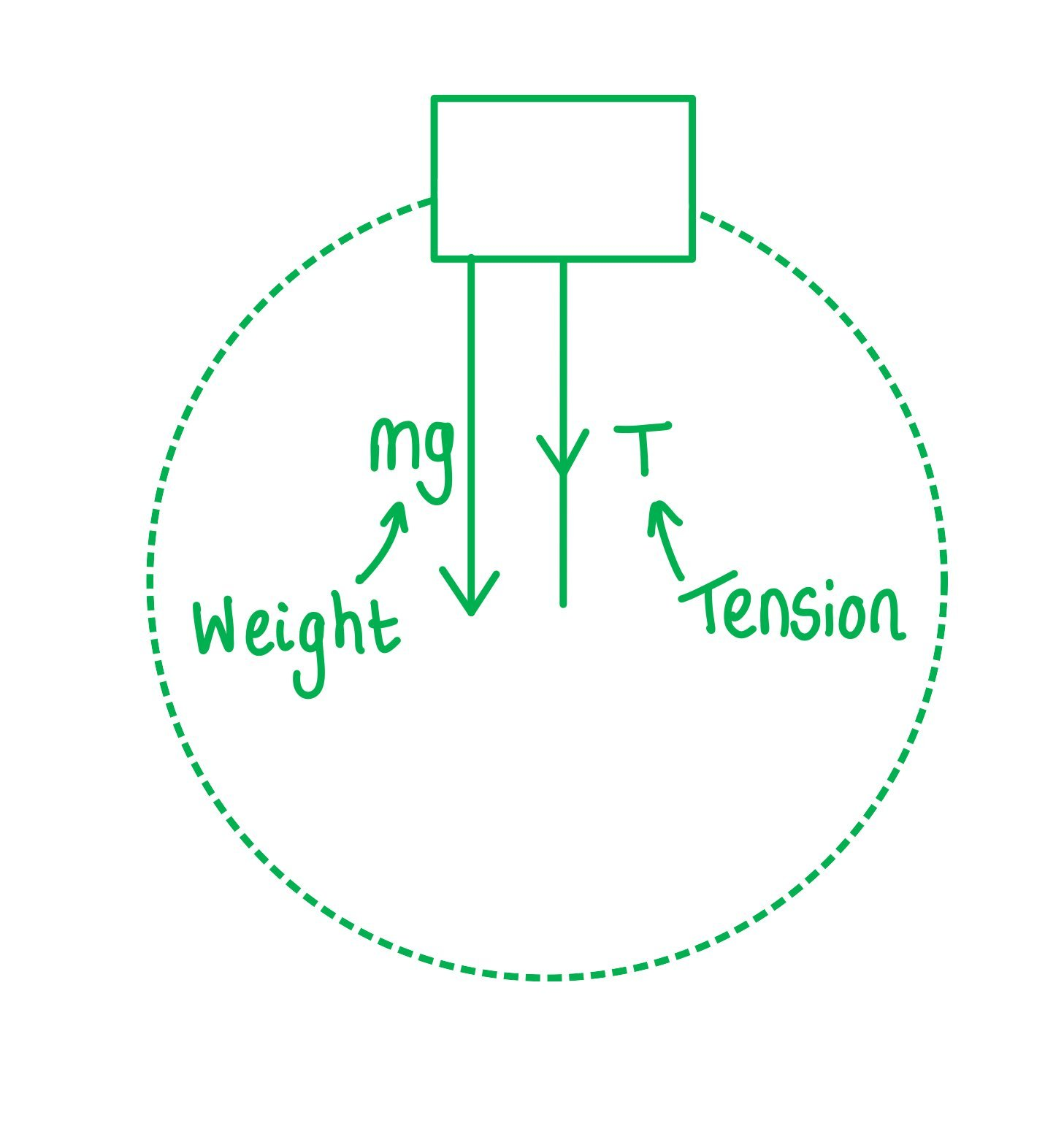# IB DP Physics: HL复习笔记6.1.2 Centripetal Force

### Centripetal Force

• An object moving in a circle is not in equilibrium, it has a resultant force acting upon it
• This is known as the centripetal force and is what keeps the object moving in a circle
• The centripetal force (F) is defined as:

The resultant force perpendicular to the velocity, and therefore directed towards the centre of the circle, required to keep a body in uniform circular motion

•  The magnitude of the centripetal force F can be calculated using:Centripetal force is always perpendicular to the linear velocity (i.e., the direction of travel)

• Where:
• F = centripetal force (N)
• v = linear speed (m s1)
• ⍵ = angular speed (rad s−1)
• r = radius of the orbit (m)
• Note: centripetal force and centripetal acceleration act in the same direction
• This is due to Newton's Second Law
• The centripetal force is not a separate force of its own
• It can be any type of force, depending on the situation, which keeps an object moving in a circular path
• For example, tension, friction, gravitational, electrical or magnetic

Examples of centripetal force• When solving circular motion problems involving one of these forces, the equation for centripetal force can be equated to the relevant force equation
• For example, for a charged particle travelling in a circle, the centripetal force causing the charged particle to move in a circle is provided by the magnetic force
• Therefore, equating the expressions for centripetal force and magnetic force gives the following:• Where:
• B = magnetic field strength (T)
• q = charge on the particle (C)
• m = mass of the particle (kg)
• v = speed of the particle (m s−1)
• r = radius of orbit (m)

A bucket of mass 8.0 kg is filled with water is attached to a string of length 0.5 m.What is the minimum speed the bucket must have at the top of the circle so no water spills out?Step 1: Draw the forces on the bucket at the topStep 2: Calculate the centripetal force

• The weight of the bucket = mg
• This is equal to the centripetal force since it is directed towards the centre of the circleStep 3: Rearrange for velocity v

• m cancels from both sidesStep 4: Substitute in values